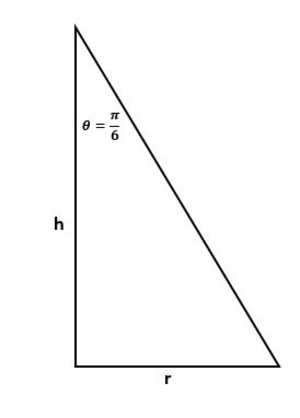# Sand falls from a hopper at a rate of 0.1 cubic meters per hour and forms a conical pile with the...

## Question:

Sand falls from a hopper at a rate of 0.1 cubic meters per hour and forms a conical pile with the side of the cone making an angle of {eq}\frac{\pi}{6}{/eq} radians with the height. At what rate is the radius increasing when the height is 3 meters?

## Time Rates:

The problem is about time rates and we are asked to find the rate that the radius is increasing givene the height. Here, we need to use the formula for volume of for a cone which is {eq}V=\frac{1}{3}r^{2}h {/eq} where {eq}h {/eq} is the height and {eq}r {/eq} is the radius. We need to express {eq}h {/eq} the height in terms of {eq}r {/eq} radius using ratio an proportion or using trigonometric funtion.

## Answer and Explanation:

Below is the graph,From the graph, by using trigonometric function,

{eq}\tan \frac{\pi }{3}=\frac{r}{h} {/eq}

{eq}h=\frac{r}{\tan \frac{\pi }{3}} {/eq}

{eq}h=\sqrt{3}r\rightarrow (1) {/eq}

The volume of the conical sand is,

{eq}V=\frac{\pi }{3}r^{2}h\rightarrow (2) {/eq}

Substituting (1) to (2),

{eq}V=\frac{\pi }{3}r^{2}\left ( \sqrt{3}r \right ) {/eq}

{eq}V=\frac{\pi\sqrt{3} }{3}r^{3} {/eq}

Differentiating with respect to {eq}t {/eq}

{eq}\frac{dV}{dt}=\frac{\pi \sqrt{3}}{3}\left ( 3r^{2}\frac{dr}{dt} \right ) {/eq}

Note that {eq}\frac{dV}{dt}= 0.1 {/eq}

Thus,

{eq}0.1=\pi \sqrt{3}r^{2} \frac{dr}{dt} {/eq}

Then, since {eq}r=\frac{h}{\sqrt{3}} {/eq}

{eq}0.1=\pi \sqrt{3}\left ( \frac{h}{\sqrt{3}} \right )^{2} \frac{dr}{dt} {/eq}

{eq}0.1=\frac{\pi}{\sqrt{3}} h^{2} \frac{dr}{dt} {/eq}

When {eq}h=3 {/eq}

{eq}\frac{dr}{dt}\approx 0.00613\:m/hr {/eq}

#### Learn more about this topic:Differential Calculus: Definition & Applications

from Calculus: Help and Review

Chapter 13 / Lesson 6
18K